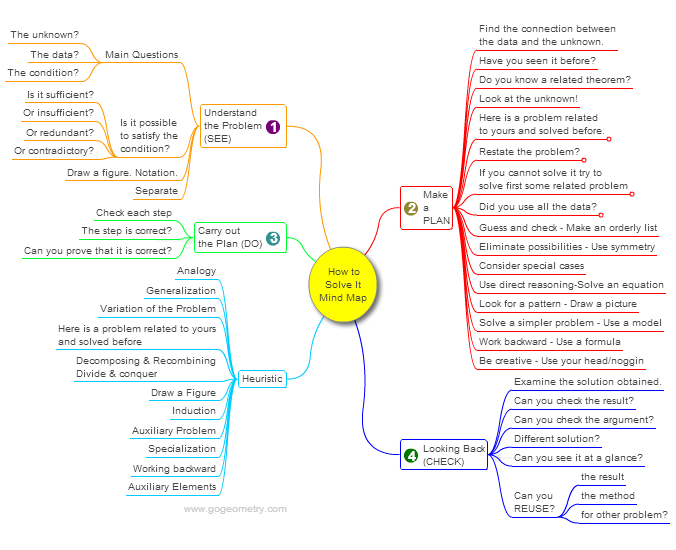# Online Geometry Problem 1076: Square, 45 Degrees, Angle, Sum, Distance, Auxiliary Construction. Level: High School, Honors Geometry, College, Mathematics Education

< PREVIOUS PROBLEM  |  NEXT PROBLEM >

In the figure ABCD is a square and the measure of angle EAF is 45 degrees. Prove that EF = BE + DF.## Mind Map: George Pólya's 1945 book, "How to Solve It"

George Pólya's 1945 book "How to Solve It, A new aspect of Mathematical Method", is a book describing methods of problem solving. It suggests the following steps when solving a mathematical problem: (1) First, you have to understand the problem. (2) After understanding, then make a plan. (3) Carry out the plan. (4) Look back on your work. How could it be better?
A mind map is a diagram used to visually organize information, knowledge, concepts or ideas.Home | Search | Geometry | Problems | All Problems | Open Problems | Visual Index | 10 Problems | 1071-1080 | Triangle | Square | 45 Degrees | Email | By Antonio Gutierrez Solution / Comment to Geometry Problem 1076 Last updated: Jan 21, 2015